# Hyperbolic Functions

• Author:
• Updated date:

Hyperbolic functions are related to the rectangular hyperbola, like the trigonometric functions are related to a circle. The equation for a hyperbola is x2/a2 - y2/b2 = 1. Hyperbolic functions are based on a hyperbola, where a = b = 1. Therefore the equation for this hyperbola is x2 - y2 = 1, when the hyperbola crosses the x-axis. When the hyperbola crosses the y-axis its equation is y2 + x2 = 1. The distance from the origin to a point P on the hyperbola is represented by the line D. The length of line D is the scale ratio σ = (x2 + y2) ½.

The angle θ is the angle between the x-axis and a line from the origin to point P (line D). This angle θ = arctan y/x. The hyperbolic functions are related to the angle θ, and are sometimes written as sinh θ or cosh θ . However the numerical value in a hyperbolic function for θ is not the value of the angle θ but the function u. For the hyperbola the function u = ln(x+y) = ln(x+(x2-1) ½).

In figure 1 we see the hyperbola and two points [P (x,y), P' (x,-y)] on the hyperbola. The area 0PaP' (the yellow area) is labeled u. The equation for this area is u = LN(x + y) = LN(x + (x2 - 1) ½). LN or ln is the natural logarithm loge based on the number e. All of the hyperbolic functions can be expressed in values of eu (see table 2 at end of this article). The hyperbolic functions are listed in table 1. On the x,t Minkowski diagram we substitute t for y.

The hyperbolic functions are listed in table 1. On the x,t Minkowski diagram we substitute t for y. In table 1 the equations shows the x,y functions equal to the x,t functions of the Minkowski diagram. On the Minkowski diagram the angle θ is equal to the arctan v/c, where v is the object's velocity. The angle θ is usualy measured between the observer's time axis t and the object's time axis t'.

## Table 1 Hyperbolic functions

Function ......Reciprocal ......Equation .............Value at 0.6c

sinh u ..........1/csch u ...........y = γv/c .......................0.75

cosh u .........1/sech u ..........x = γ .............................1.25

tanh u ..........1/coth u ........y/x = (γv/c)/(γ) = v/c ......0.6

coth u ..........1/tanh u ........x/y = c/v .........................1.667

sech u .........1/cosh u .......1/x = 1/γ .........................0.8

csch u .........1/sinh u .........1/y = c/γv ......................1.333

Angle θ = arctan y/x = arctan v/c

u = LN(x+y) = LN(x+(x2-1)½) ................where γ = 1/(1-v2/c2)1/2

Example: In fig. 1 for a point P on the hyperbola, x=1.25 and y=0.75 then u = ln(x+y) = ln(2) = 0.693147 and using a table or calculator, we find that sinh u = 0.75, cosh u = 1.25 and tanh u = 0,6. The relationship between θ and u can be seen in tan θ = y/x.

Sometimes sinh u is written as sinh θ that relates the function to the angle and sometimes it is written as sinh x. However, all these represent sinh ln(x+y).

## The hyperbolic functions and their relationship to the attributes of relativity on the Minkowski diagram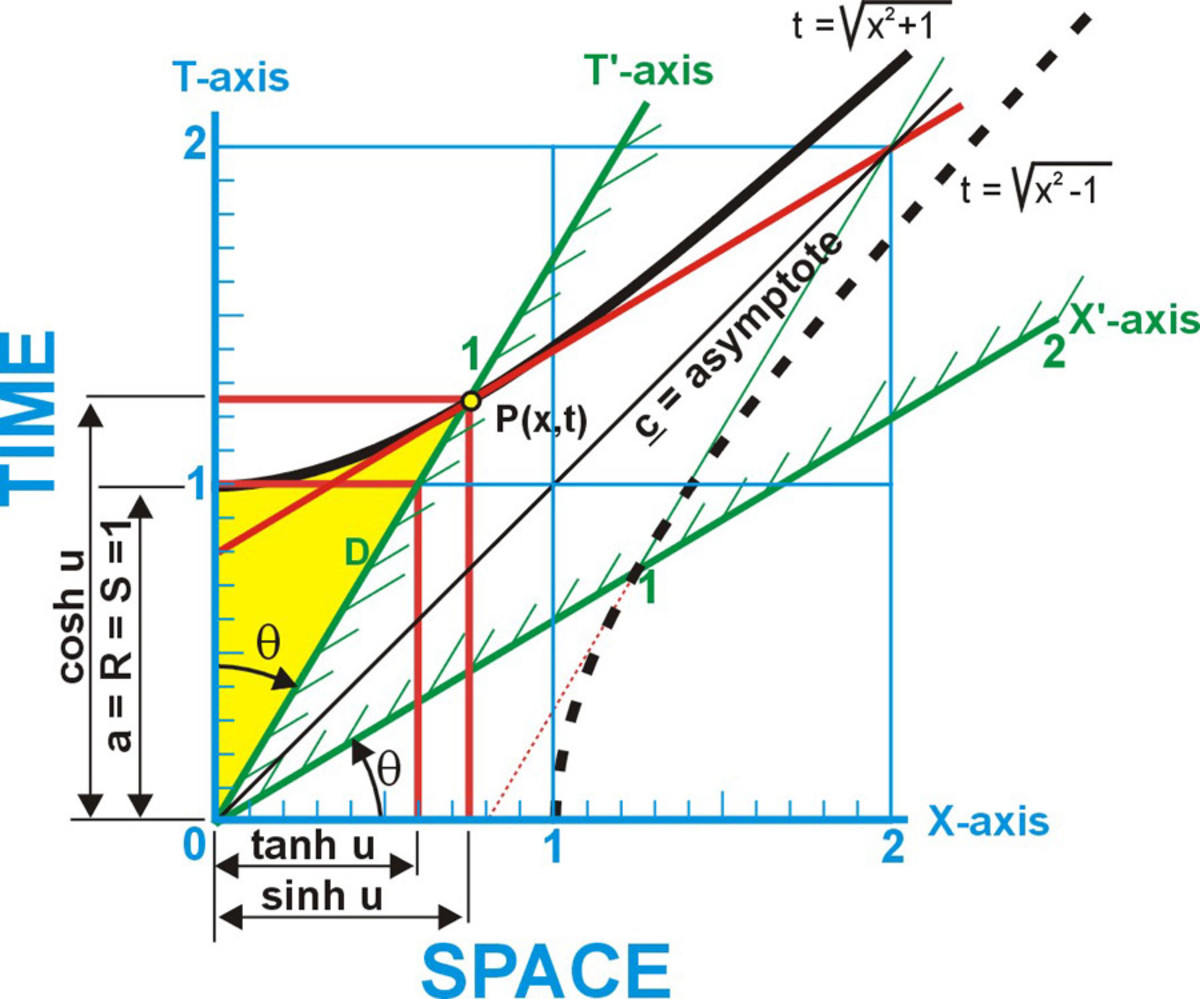Fig. 2 Fundamental hyperbolic functions of the time hyperbola

In figure 2 and 3 we see the Minkowski diagram. The time hyperbola, the hyperbola that crosses the time axis, is drawn in a soild black line. The space hyperbola is shown as a dotted black line. The qualities of the time hyperbolic functions are represented for an object with a relative velocity of 0.6c to observer. On the Minkowski diagram the angle θ is the angle between the observer's time axis T and the object's time axis T'. The dimension for each hyperbolic function for an object with this velocity is shown in brackets in the observer's space units SU or time units TU.

sinh u = x = γ(v/c) = The observer's distance the object travels in 1 of object's time units. [0.75 SU].

cosh u = t = γ = Time dilation, the object's time unit. The proportional of mass or energy increase with relative velocity. [1.25 TU].

Scroll to Continue

tanh u = x/t = v/c = the relative velocity of the object and the distance the object travels in 1 observer's time unit [0.6 SU]. This also represents the offset between two clocks one space unit apart. The trailing clock will v/c ahead of the leading clock.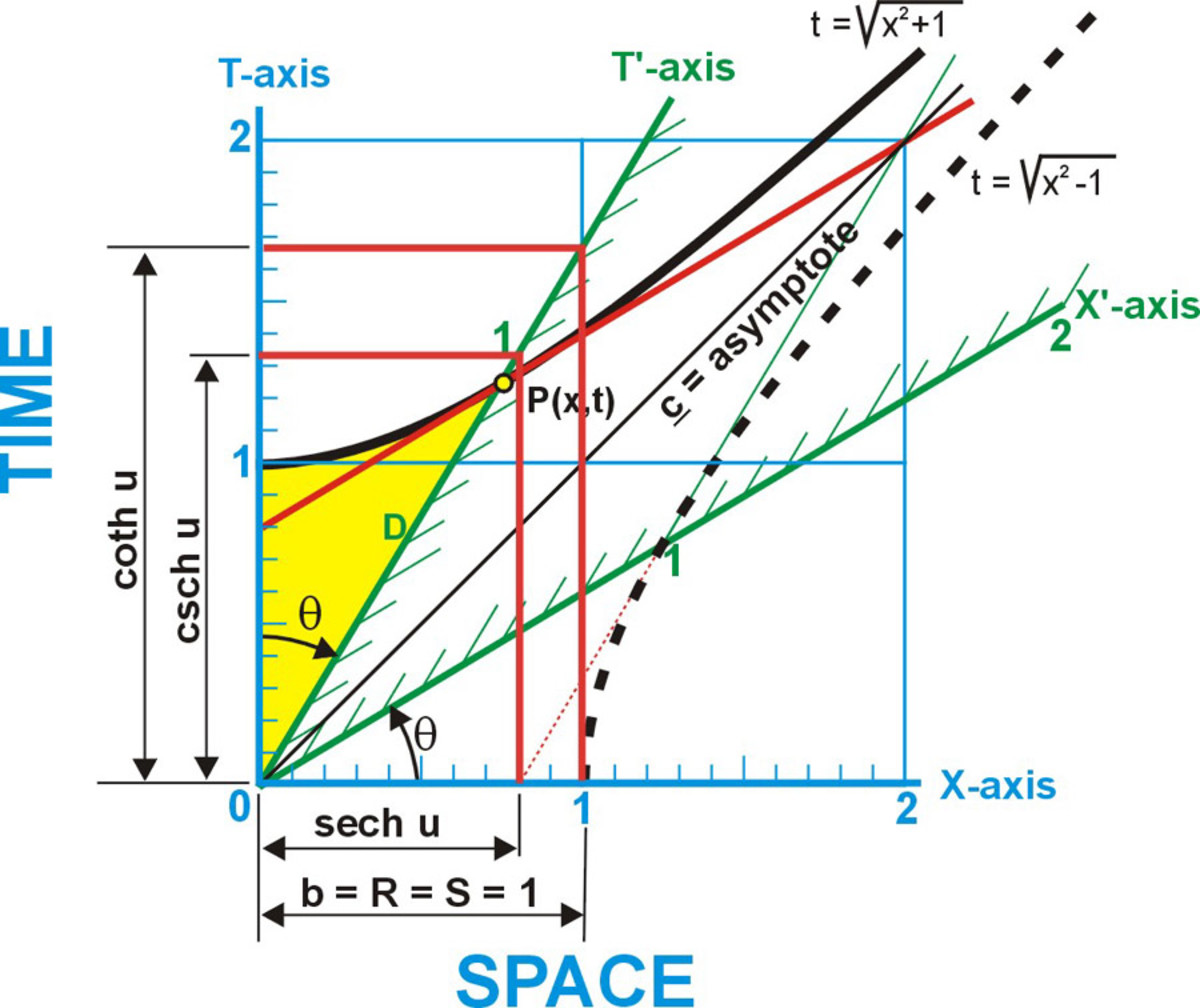Fig. 3 Reciepocal hyperbolic functions of the time hyperbola

coth u = t/x = c/v = the time in observers time units it takes the object to travel 1 of the observer's space units. [1.666...TU]

sech u = 1/t =1/γ The amount of spatial contraction, the horizontal distance through one of the object's space units. [0.8 SU]

csch u = 1/x = (c/v)/γ = The time it takes the length of the object (1unit long in its own system) to pass a point in the observer's system. [1.333...TU]

Here we see that all of the attributes of relativity can be illustrated on the Minkowski diagram as hyperbolic functions of the time hyperbola.

## The hyperbolic functions and their relationship to the attributes of the DeBroglie wave on the Minkowski diagram

In fig, 4 and 5 the hyperbolic functions of the space hyperbola are represented for an object with a relative velocity of 0.6c to observer. The t' axis has a slope of v/c = 0.6 representing the speed of the object as 0.6c. The DeBroglie wave, the wave associated with particles of matter, has a velocity c2/v. On the diagram this velocity would be represented by (c2/v)/c = c/v. The x' axis has a slope of c/v to the x-axis. Arctan c/v is the angle Φ. Could it be that the slope of the x' axis represents the velocity of a DeBroglie like group wave. To see if the hyperbolic functions could indicate the attributes of the DeBrglie wave, consider that the wavelength of the original electromagnetic wave were the Compton wavelength for a particle of matter. If we were to divide the equation for the DeBroglie wavelength

= h/p = h/mv = h/γmOv) ........ where γ = 1/(1-(v/c)2)1/2

by the equation for the Compton wavelength

λO = hc/mOc2 = h/(mOc)

for the same particle, we obtain a ratio between the two wavelengths. That is the Wavelength ratio.

λ/λO = (h/γmOv)/( h/mOc) = (c/v)/γ.

By using the observer's one space unit as the Compton wavelength we can use the ratio to illustrate the DeBrolie wavelength on the Minkowski diagram. We can do the same with all of the attributes of the DeBroglie wave for the same particle of matter with a velocity of v. This way all of the attributes of the DeBroglie wave can be illustrated on the Minkowski diagram using those ratios. These ratios are explained in more detail in the article "What could cause the effects of relativity".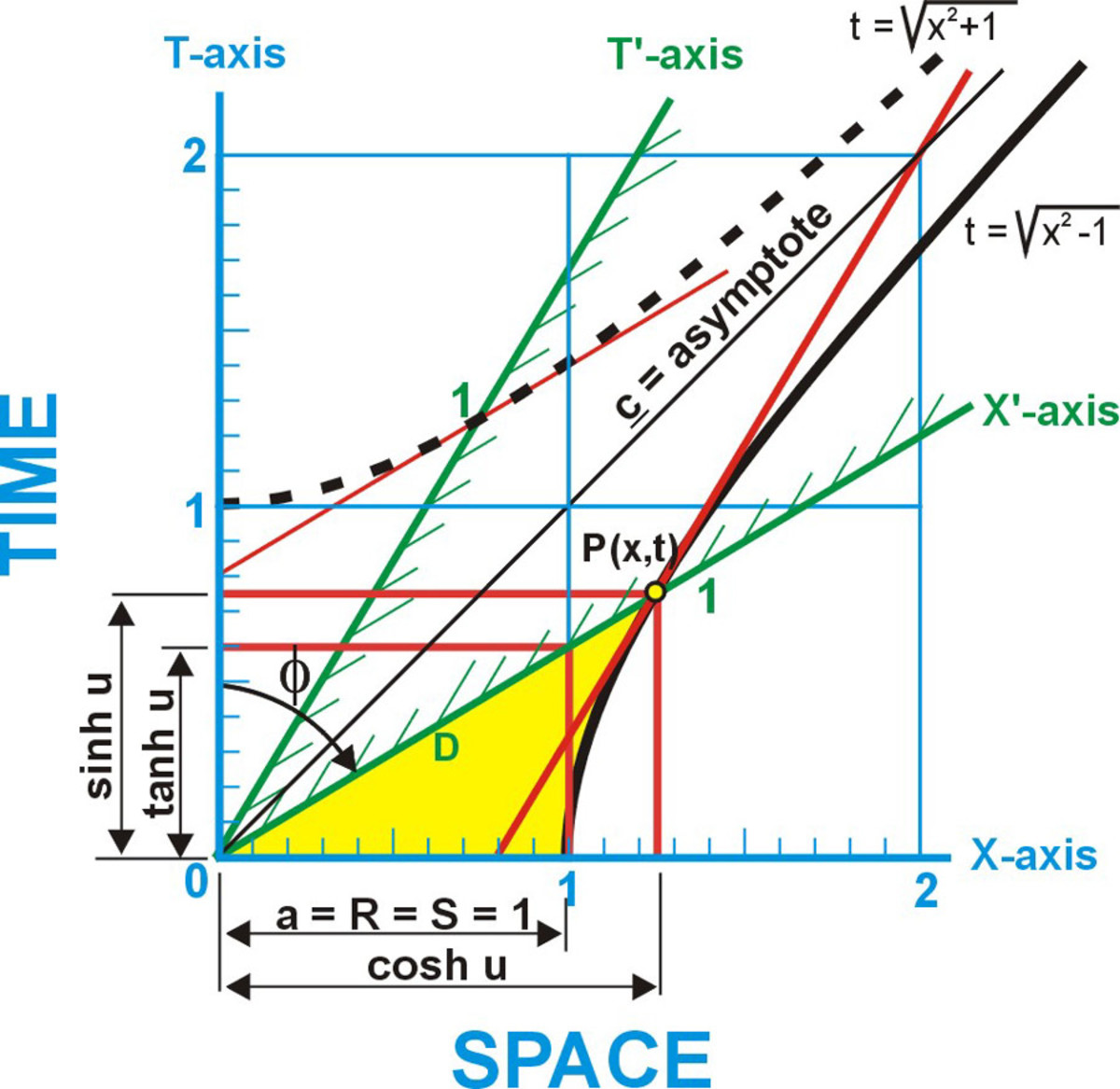Fig. 4 Fundamental hyperbolic functions of the space hyperbola

In figure 4 and 5 the qualities of the time hyperbolic functions are represented for an object with a relative velocity of 0.6c to observer

sinh u = t = γ/(c/v) = The time it takes the deBroglie wave to travel one of the object's space units (1.25/1.66...). The time period of DeBroglie wave/time period of Compton wave [0.75 TU]

cosh u = x = γ = The distance the deBroglie wave would travel in 0.75 observer's time units. vt; (c/v)[ γ/(c/v)] = γ = the frequency of the DeBroglie wave divided by the Compton frequency [1.25 SU]

tanh u = 1/(c/v) = v/c the time it takes the deBroglie wave to travel one of the observer's space units [0.6 TU]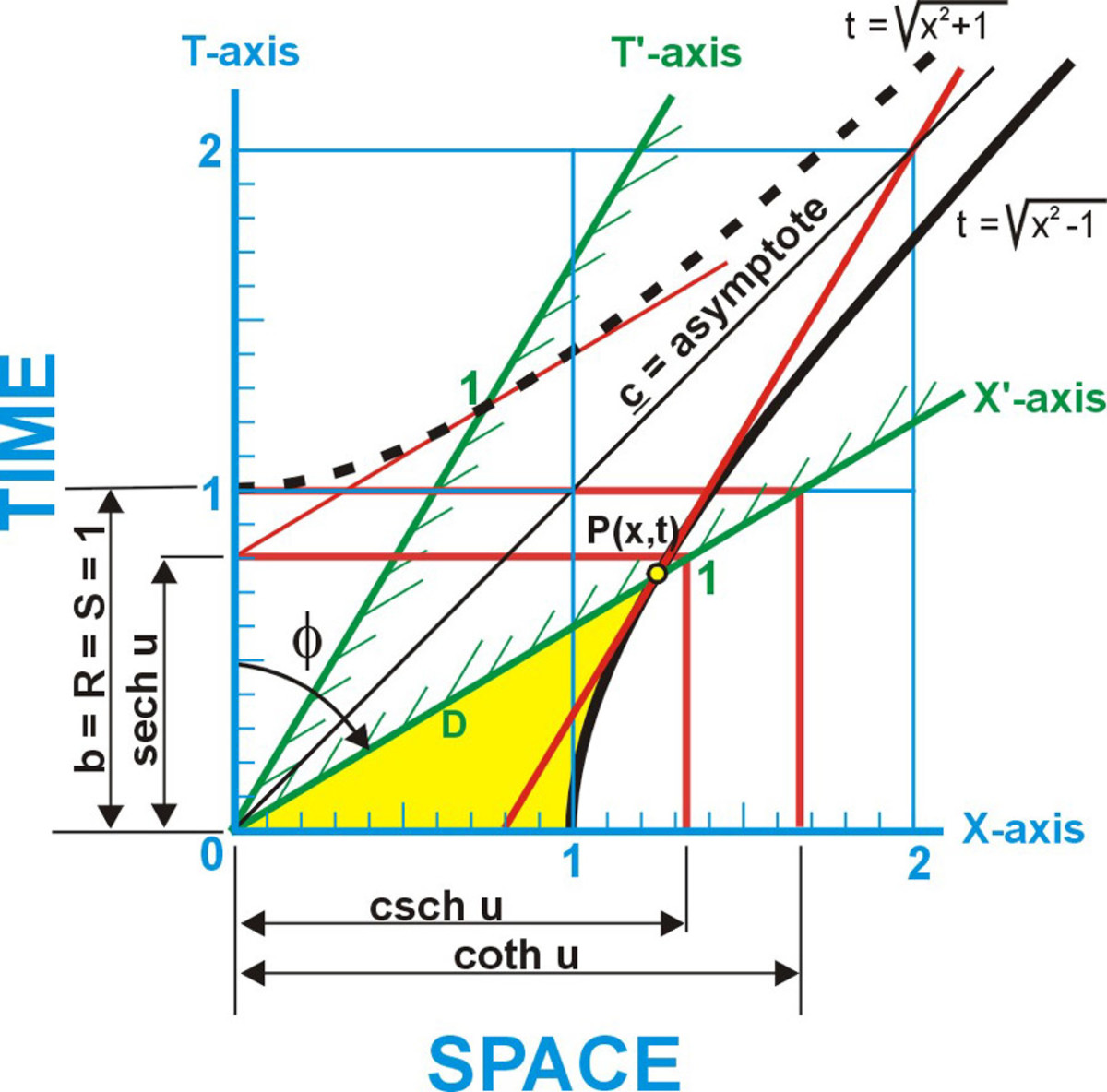Fig. 5 Reciepircal hyperbolic functions of the space hyperbola

coth u = t(c/v) = 1(c/v) = c/v = The velocity of the DeBroglie wave. The distance DeBroglie wave travels in 1 of observer's time units [1.666...SU]

sech u = 1/x =1/γ = The time it takes the DeBroglie wave to move the distance of one DeBroglie wavelength. [0.8 TU]

csch u= 1/t = (c/v)/γ = The DeBroglie wavelength divided by the Compton wavelength [1.333...SU]

Here we see that all of the attributes of the DeBroglie wave can be illustrated on the Minkowski diagram as hyperbolic functions of the space hyperbola.

The equation for a circle with a radius of one unit is y = (1-x2)1/2. If x<-1 or x>1 then the equation results in the square root of a negative number. The square root of a negative number is not a real number. However, the square root of any negative number can be factored into square root of a positive number times the square root of negative one. The square root of minus one (-1) 1/2 is called an imaginary number and is designated by the letter i. Thus the square root of -4 = 2i. In a coordinate system 2i is a complex number x + yi. We can not plot the complex numbers containing x and yi on the x,y Cartesian plane*. However these complex numbers could be plotted on a Gaussian number plane which is 90O to the real (x,y) plane. In figure 2, to relate this plane to the Minkowski diagram the yi axis becomes the cti axis. When -1<x<1 the circle equation draws a circle on the real (x,y) plan. When x<-1 or x>1 and the equation for the circle is plotted on the Gaussian number plane it draws a hyperbola.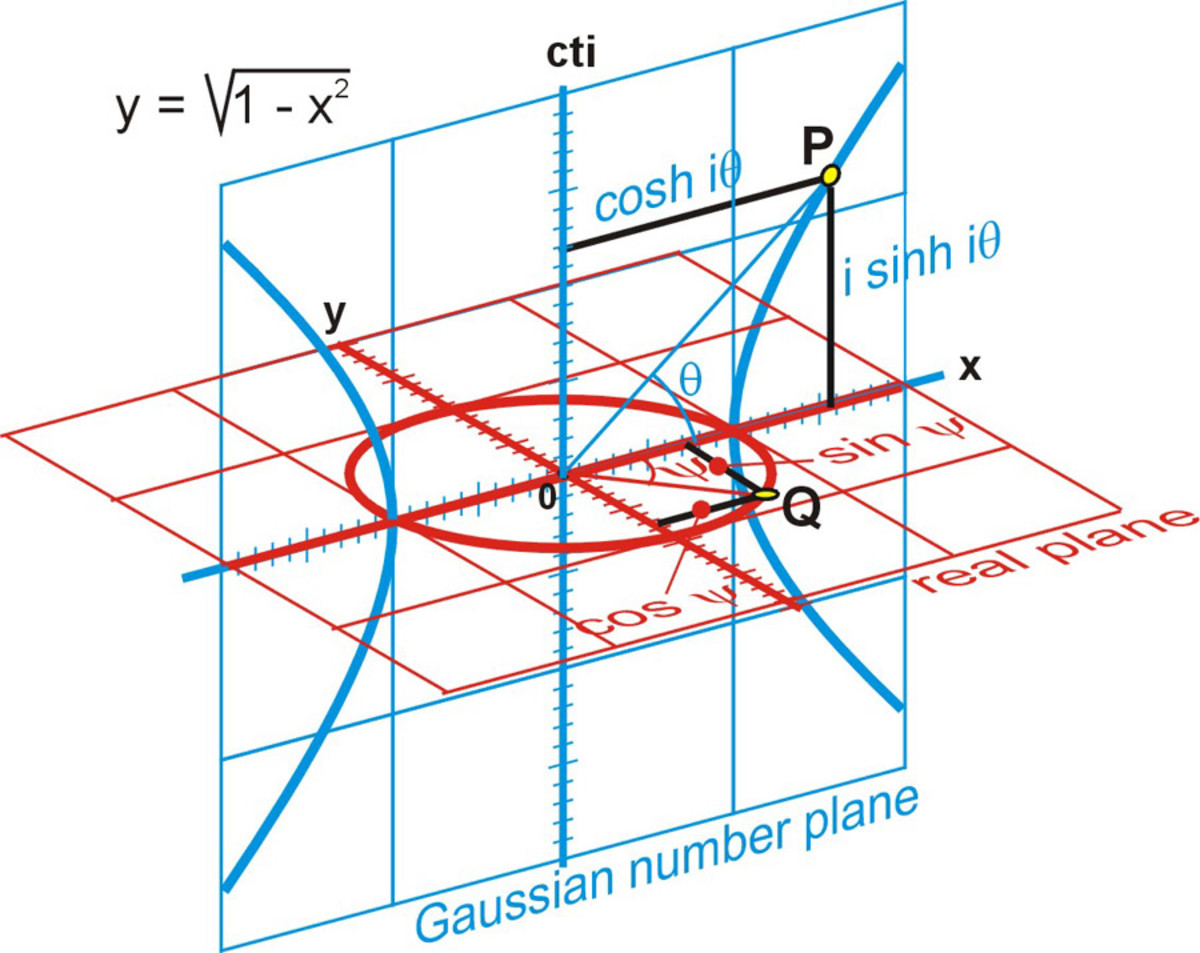Fig. 6 The hyperbola of the space axis

In fig. 6 we see the hyperbola of the space axis of the x,t Minkowski diagram. We see the angle between the x-axis and a line from the origin and a point P on the hyperbola is θ or iθ. We see the distance from the x-axis to a point P on the hyperbola is the i sinh iθ.

The distance from the cti-axis to a point P on the hyperbola is the i cosh iθ We see the angle between the x-axis and a line from the origin and a point Q on the circle is ψ or iψ. We see the distance from the x-axis to a point Q on the circle is the sin ψ. The distance from the y-axis to a point Q on the circle is the cos ψ

In fig. 7 a special relationship occurs between this circle and this hyperbola when the cos ψ (n) of the circle equals the reciprocal of the cosh θ (1/n) of the hyperbola. The dimensions of all six different circular functions are equal to the dimensions of all six different hyperbolic functions. Then the circle and the hyperbola are said to be related by the Gudermannian function. The definition of the Gudermannian is the function ψ of the variable θ satisfying tan ψ = sinh θ or sin ψ = tanh θ. Where ψ is the Gudermannian of θ is written gdθ.* In other words, the circular and hyperbolic functions can be related by the Gudermannian function when the length of the sine of the circle is equal to the length of the tangent (tanh) of the hyperbola.*

Fig. 7 illustrates all of circular functions compared to the hyperbolic functions related by the Gudermannian function. These are arranged into 3 sets where one circular function and its reciprocal are equal to a hyperbolic function and its reciprocal. To relate these functions to the Minkowski diagram, the angle θ of the hyperbolic functions is the arctan v/c and the angle ψ of the circular functions is the arcsin v/c.

In A of fig. 7 cos ψ = n the x distance to point Q on the circle. In these drawings v/c = 0.6c and n = (1-(v/c)2)1/2 = 0.8. Cosh θ = 1/n = 1/0.8 = 1.25, the x distance to the point P on the hyperbola. Sec ψ = cosh θ = 1/n =1/0.8 = 1.25. This is the hypotenuse of a right triangle whose sides are 1 and cot ψ. Sech θ = n = 0.8.

In B of fig. 7 sin ψ = (1-n2)1/2 = 0.6 the y distance to point Q on the circle. The distance along the line from the origin through point P to y=1 is the csc ψ = 1/(1-n2)1/2 = 1.6666. Tanh θ is the tangent to the hyperbola at the point where the x-axis crosses the curve. Tanh θ = (1-n2)1/2 = 0.6 the length of one side of a right triangle whose other side is one unit and opposite the angle θ. Coth θ is the tangent to the hyperbola at the point P on the curve. Coth θ = 1/(1-n2)1/2 the length of one side of a right triangle whose other side is one unit and opposite the angle Φ. The angle Φ is the compliment of angle θ. Angle Φ = 90O- θ = arctan c/v. The length of the coth θ is illustrated here as a horizontal line when the opposite side cti =1, and also as a vertical line when the opposite side x = 1.

*Mathematics Dictionary by James and James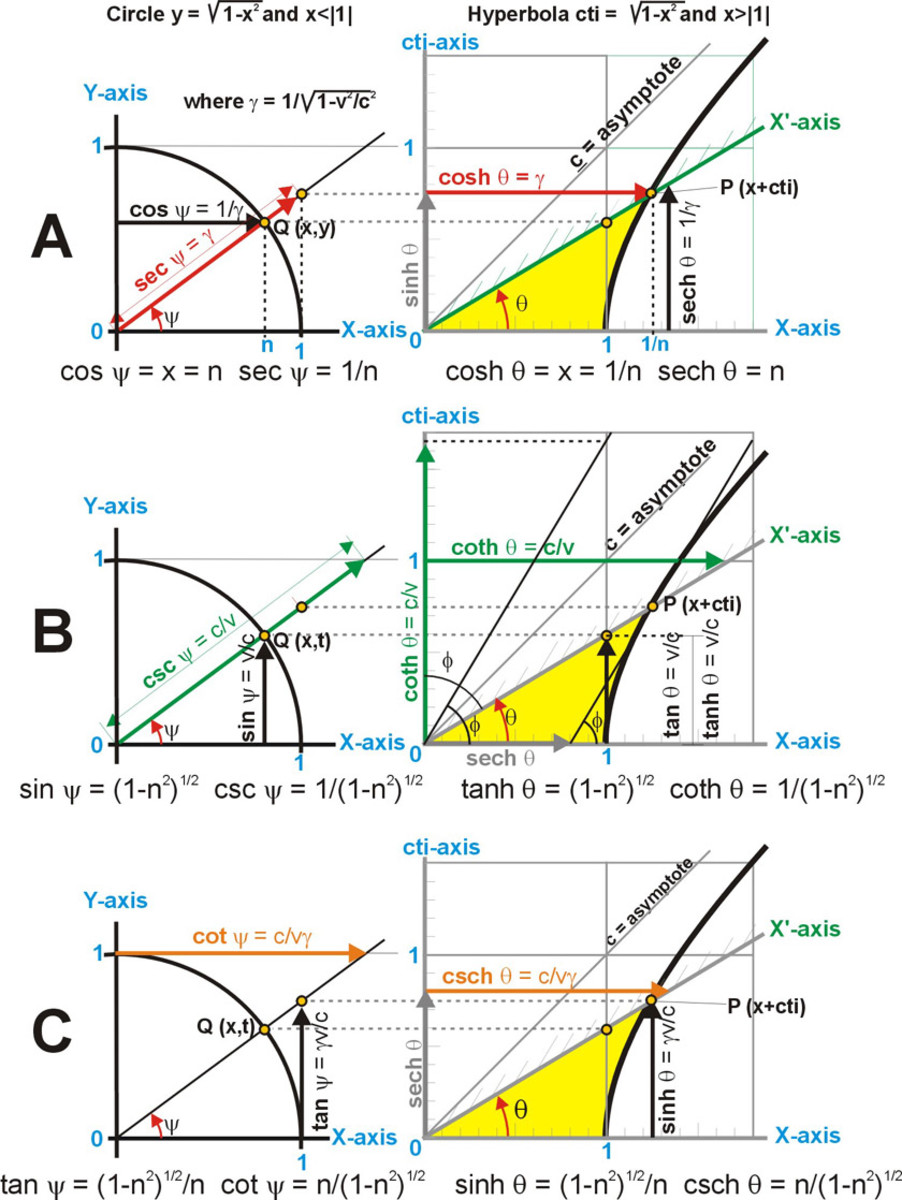Fig. 7 Comparing the circle with the hyperbola using the Gudermannian relationship

In C of fig. 7 tan ψ = (1-n2)1/2/n = 0.75 the length of one side of a right triangle whose other side is one unit and opposite the angle Ψ. Cot Ψ = n/(1-n2)1/2 = 1.333, the length of one side of a right triangle whose other side is one unit and opposite the angle 90O-ψ. Sinh θ = 0.75 is the cti distance to the point P on the hyperbola. Csch θ = n/(1-n2)1/2 = 1.333, the length of one side of a right triangle whose other side is sech q and opposite the angle 90O

## e (epsilon) the natural number

The constant e (which is the initial letter in the word exponential and is sometimes called Euler's number) is the limiting value of the fraction (1 + 1/n)n as n approaches infinity. The value of e may be found to as many places as desired by expanding the fraction (1 +1/n) n by the binomial theorem and letting n approach infinity. Figure 8 shows the curves for the prime hyperbolic functions produced by adding exponential curves.

e = lim(1 + 1/n)n = 1 +1/1! + 1/2! + 1/3! +1/4! + 1/5!.... 1/n!

= 2 + 1/2 + 1/6 +1/24 +1/120 + 1/720 + 1/5040 + .... = 2.7182818284 ...

## Table 2 Exponential Definitions of Hyperbolic functions

sinh u = y/a = ½(eu-e-u)

cosh u = x/a = ½(eu+e-u )

tanh u = y/x = (eu-e-u )/(eu+e-u ) = sinh u/cosh u

coth u = x/y = (eu+e-u )/(eu-e-u ) = cosh u/sinh u

sech u = a/x = 2/(eu+e-u ) = 1/cosh u

csch u = a/y = 2/(eu-e-u ) = 1/sinh u

Example: For a point on a hyperbola at x = 1.25 and y = 0.75

½ eu = ½ e ln(1.25+0.75) = ½ eln2 = ½ (2) = 1

½ e-u = ½ e -ln(1.25+0.75) = ½ e ln½ = ½(½) = 0.25

sinh u = 1 - 0.25 = 0.75 and cosh u = 1 + 0.25 = 1.25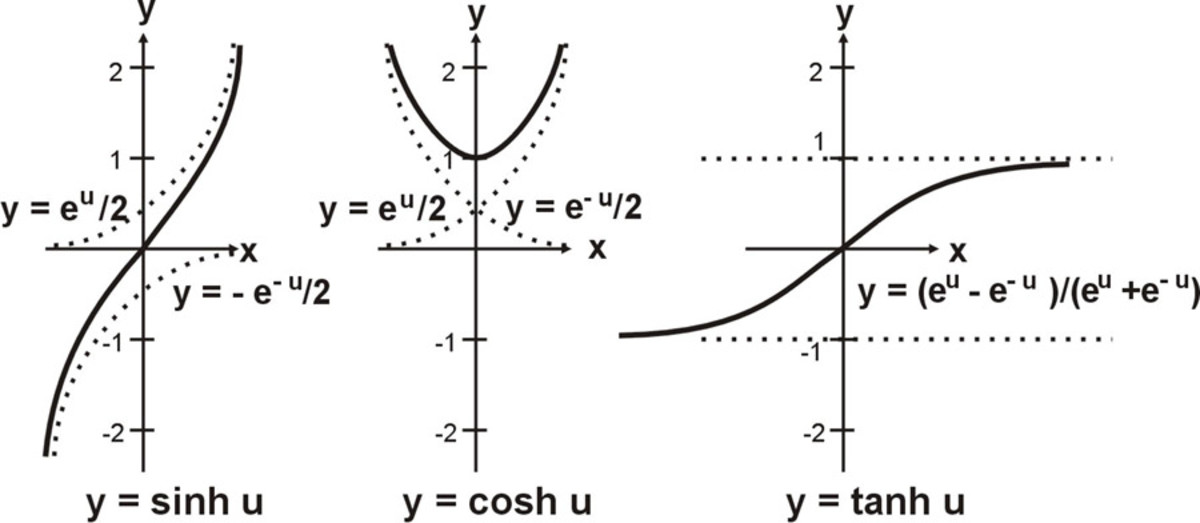Fig. 8 The relationship between e and the hyperbolic functions*

We have seen the hyperbolic functions and their relationship to the x,t Minkowski diagram. The hyperbolic functions and their relationship can be compared to the attributes of relativity and the DeBroglie wave. Comparison of circular and hyperbolic functions can be related by the gudermannian function and i the imaginary number. We saw the relationship between e and the hyperbolic functions.

* Analytic Geometry with Vectors by Douglas Riddle

P. K. Shee on March 29, 2012:

Your handling of the complex theme is lucid and masterly.

Young Shin on August 27, 2010:

Hi Blog master

I really appriciate for your wondful work in this site. I have searched many sites but i couldn't find any site which provides me more fundamental information for the subject than your site.

I also really enjoy finding the hidden part of this function from your work.

Many thanks

hyperb on September 21, 2009:

Can you express i*csc() in the real domain?